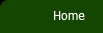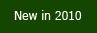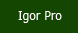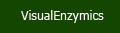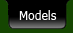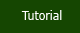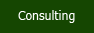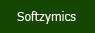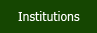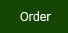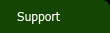VisualEnzymics offers analysis for nine types of enzyme binding and kinetic data, and includes 98 model equations: one substrate rate saturation data one substrate one inhibitor data pH rate profiles exponential data dose response data two substrate data one substrate one activator data binding data tight binding data Each analysis module holds up to 10 separate data sets and each module has its own graph window with specially formatted graphs that match the type of analysis. All data sets can be graphed individually, or multiple data sets can be overlaid in a single graph. Estimates and fit curves are automatically updated and linked to each graph window.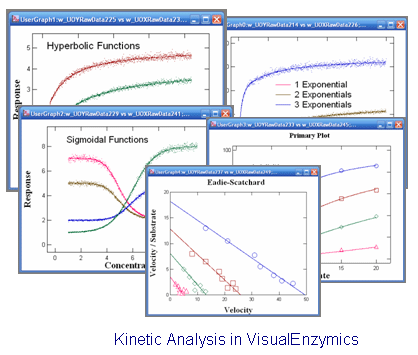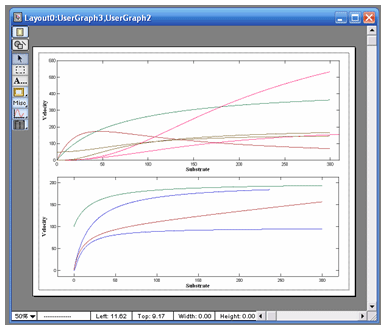## One Substrate Data

VisualEnzymics provides 10 equations for fitting steady state rate saturation profiles. These include Michaelis-Menten, Michaelis-Menton plus offset, Michaelis-Menten plus linear phase, Hill, Hill plus offset, substrate inhibition, two site, two/one, sigmoid, and cubic.  These equations describe a variety of hyperbolic and sigmoidal saturation profiles, and can be fitted to almost any type of rate saturation data. The equations all contain two, three, or four parameters. Parameters can be floated or individually held constant during fitting. Data can be weighted four different ways, including constant weighting, proportional weighting, between constant and proportional, or by standard deviation. You can choose one of four different fitting algorithms, including Levenberg-Marquardt, Levenberg-Marquardt Robust, Monte Carlo, and Monte Carlo Robust.

## One Substrate One Inhibitor Data

VisualEnzymics provides nine equations for fitting steady state inhibition data. These include competitive, noncompetitive, uncompetitive, hyperbolic competitive, hyperbolic noncompetitive, hyperbolic uncompetitive, sigmoidal competitive, sigmoidal noncompetitive, and sigmoidal uncompetitive. These equations will fit inhibition mechanisms for Michaelis-Menten type enzyme kinetics, and for enzymes displaying cooperative behavior. Enter data for an inhibition experiment as substrate concentration, inhibitor concentration, velocity, and standard deviation of the velocity. VisualEnzymics will automatically parse the data according to inhibitor concentration, and plot your results as groups of data at each inhibitor concentration. One click buttons will generate automatic data transforms to Lineweaver-Burk, Hanes-Woolf, Woolf-Hofstee, or Eadie-Scatchard formats. Graphs can be exported in 5 different graphic formats for electronic presentations or publication in journals.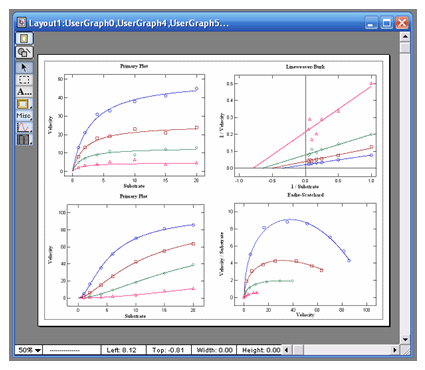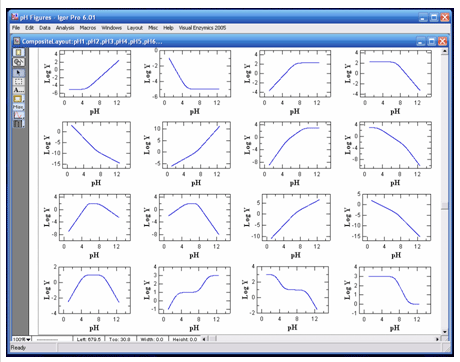## pH Data

VisualEnzymics provides 16 pH rate profile equations for fitting the pH dependence of binding or kinetic parameters. All enzymes and proteins are sensitive to protons, and the variation of reaction parameters as a function of pH yields information about the titratable groups that participate in binding and catalysis. These reactions may involve acidic or basic groups, and may yield various curve shapes as a function of pH. VisualEnzymics provides equations describing single or multiple inflection point pH titration curves. Data may be plotted as Y versus pH, or Log Y versus pH. Since pH dependence plots typically involve parameter variation over several orders or magnitude, VisualEnzymics offers proportional weighting to achieve more accurate fits to data at the extreme of the pH profile. Data may be fitted with either the Levenberg-Marquardt or Monte Carlo fitting algorithms. Multiple data plots can be overlaid on the same graph to compare data from different experiments. Plots can be combined in page layouts for publication or lab notebooks.

## Exponential Data

VisualEnzymics provides 24 equations for fitting exponential data. There are eight equations for single exponential data plus baseline, eight equations for the sum of two exponentials plus baseline, and eight equations for the sum of three exponentials plus baseline. The exponential equations will fit any variety of curve shape that follows exponential behavior. Exponential behavior may derive from transient kinetics in the form of response versus time data, or may derive from physical processes such as radioactive decay. All fits yield the observed rate constant and the amplitude of the exponential. When the initial estimates of the rate constants are unknown, the data can be fitted by the Monte Carlo method to obtain good initial estimates. The fit then can be optimized further by using the initial estimates in the Levenberg-Marquart fitting algorithm.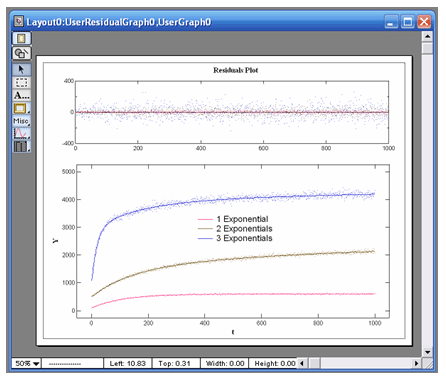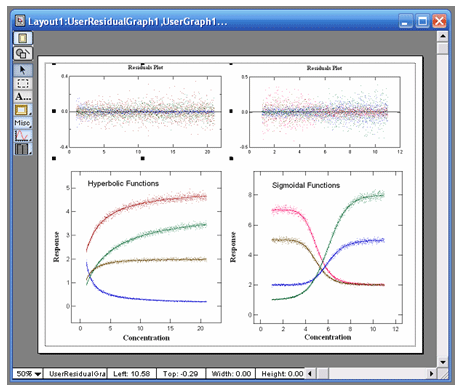## Dose Response Data

VisualEnzymics provides eleven equations for fitting dose response data. These types of equations can be used to fit activity versus ligand concentration data when the enzyme has not been purified from a more complex biochemical system, or where the response mechanism is unknown, or where the response depends on biochemical interactions beyond the enzyme itself. These equations provide three, four, and five parameter logistic fits. Logistic equations yield the minimum, maximum, half-saturation point, slope of the inflection point, and skewness of a dose dependent response. The shapes of these curves can be hyperbolic or sigmoidal in decreasing or increasing direction. Fits to these equations will yield the fitted parameters and standard error of the parameters, as well as the user-specified confidence interval.

## Two Substrate Kinetic Data

VisualEnzymics provides seven equations for fitting two substrate steady state rate saturation profiles. These include Ordered Bi Uni, Random Bi Bi, Random Bi Bi with Product Inhibition, Random Bi Bi with Substrate Inhibition, Ping Pong, Ping Pong with Competitive Substrate Inhibition , and Ping Pong with Double Competitive Substrate Inhibition. The fitting algorithm fits data at all combinations of Substrate A and Substrate B simultaneously. The graph window automatically plots data as either velocity versus Substrate A at fixed concentrations of Substrate B, or as velocity versus Substrate B at fixed concentrations of Substrate A. Data are automatically sorted by substrate concentration and are plotted as individual groups of data. Each graph can be transformed to Lineweaver-Burk, Hanes-Woolf, Woof-Hofstee, or Eadie-Scatchard formats. All graphs can be added to layouts or exported to electronic presentation programs.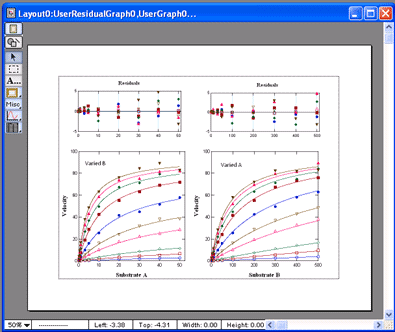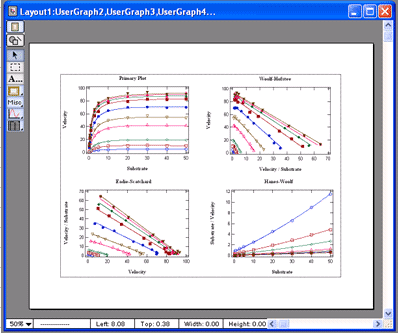## One Substrate One Activator Data

VisualEnzymics provides eight equations for fitting one substrate one activator steady state rate saturation profiles. These equations derive from models where a metal ion is the activator, and where the metal ion can combine with the enyzyme, the substrate, or both, and activate the enzyme. However, the form of the equations can represent any activator that combines to form the activated species. The models include various types of non-essential and essential activation.
The fitting algorithm fits data at all combinations of substrate and activator simultaneously. Data are automatically sorted by activator concentration and are plotted as individual groups of data. Each graph can be transformed to Lineweaver-Burk, Hanes-Woolf, Woof-Hofstee, or Eadie-Scatchard formats. All graphs can be added to layouts or exported to electronic presentation programs.

## Equilibrium Binding Data

VisualEnzymics provides eight equations for fitting equilibrium ligand binding data. These include both a simple hyperbolic one step binding equation and a quadratic equation for one step binding. The quadratic equation is available in molar binding terms and in spectroscopic terms based on the molar extinction coefficient of the bound complex. VisualEnzymics also includes two equations for one site competitive binding, which can be used to fit cases where one ligand with a measurable signal is used to displace a second ligand that has no intrinsic binding signal. The program also has three equations to fit two site binding where one ligand bindings to two sites with different affinity. The two site equation is provided in three forms for fitting to data based on the extinction coefficient, data based on fluorescence titrations, and data based on radio-ligand titrations. The competitive and two site binding equations fit to the root of a general cubic equation, and will fit hyperbolic and non-hyperbolic curve shapes. Each graph can be transformed to Lineweaver-Burk, Hanes-Woolf, Woof-Hofstee, or Eadie-Scatchard formats. All graphs can be added to layouts or exported to electronic presentation programs.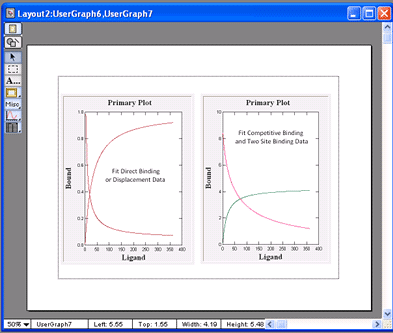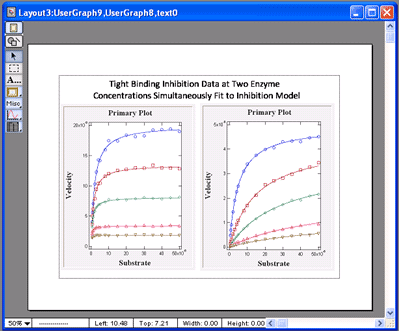## Tight Binding Kinetic Inhibition Data

VisualEnzymics provides four equations for fitting tight binding inhibition data. Tight binding inhibition occurs when the Kd for inhibitor binding is approximately the same as the enzyme concentration in the experiment. This leads to significant depletion of the free inhibitor concentration, and special quadratic equations must be used to describe the inhibition data correctly. VisualEnzymics provides the correct quadratic form of the equations for competitive, noncompetitive, uncompetitive, and mixed tight binding inhibition. Also, it provides the ability to perform global tight binding inhibition analysis on data obtained at different enzyme concentrations. Varying the enzyme concentration is the most effective way to demonstrate the presence of tight binding inhibition, and to demonstrate that the inhibition constant changes as the enzyme concentration changes. The global fitting capability in VisualEnzymics simultaneously fits the data at all enzyme concentrations, and provides the true inhibition constant for the inhibitor.